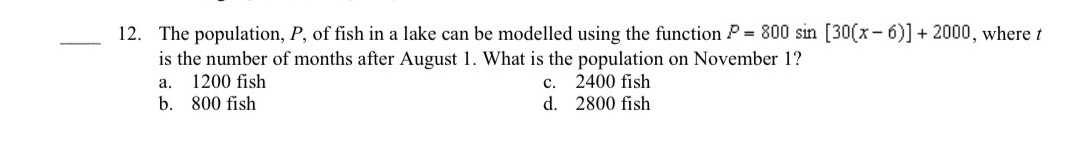### ¿Todavía tienes preguntas de matemáticas?

Pregunte a nuestros tutores expertos
Algebra
Pregunta12. The population, $$P$$ , of fish in a lake can be modelled using the function $$P = 800 \sin [ 30 ( x - 6 ) ] + 2000$$ , where $$t$$ is the number of months after August 1. What is the population on November 1? a. $$1200$$ fish c. $$2400$$ fish b. $$800$$ fish d. $$2800$$ fish